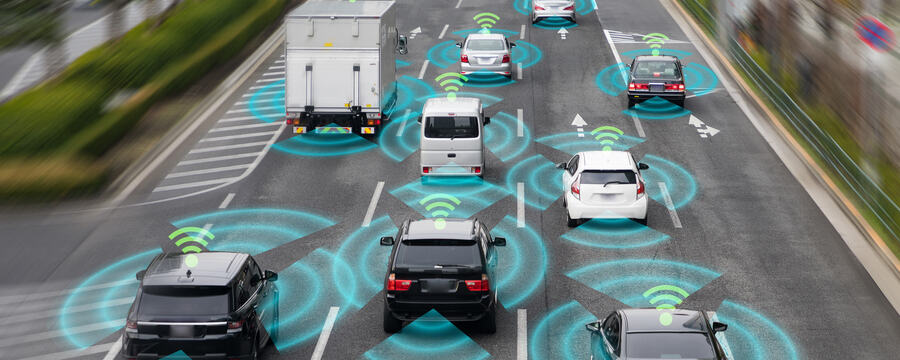New offer! Get 30% off one whole year of Unlimited learning. Subscribe for just £249.99 £174.99. New subscribers only T&Cs apply

•# Transformation of Pose in 2D

How to transform the pose of a robot from its body frame to a fixed frame? This article dives into that.

To transform a pose in 2D from the body frame {B} to the fixed frame {A}, we perform two main operations: translation and rotation. The combined operation is known as transformation.

Let t be the 2 x 1 translation vector of the robot’s body frame {B} given with respect to the fixed frame {A}. Let theta be the orientation of the robot, i.e the heading angle. Therefore, the corresponding 2 x 2 rotation matrix R obtained for the frame {B} with respect to an intermediate frame {V} is given as [cos(theta) -sin(theta);sin(theta) cos(theta)]. The elements comprising the rotation matrix are dependent on the axis of the rotation taken into account.

In the simple 2D case, the robot is constrained to rotate around its Z-axis, known as yaw. The sign of the rotation follows the right-hand rule. A homogenous matrix that considers both the rotational components and the translation components is known as the transformation matrix T. This is a 3 x 3 matrix given as [R;t;0 0 1] .

Finally, the pose of the robot in the {B}-frame, [x y theta], is transformed to {A}-frame by multiplying the transformation matrix T x [x y 1].